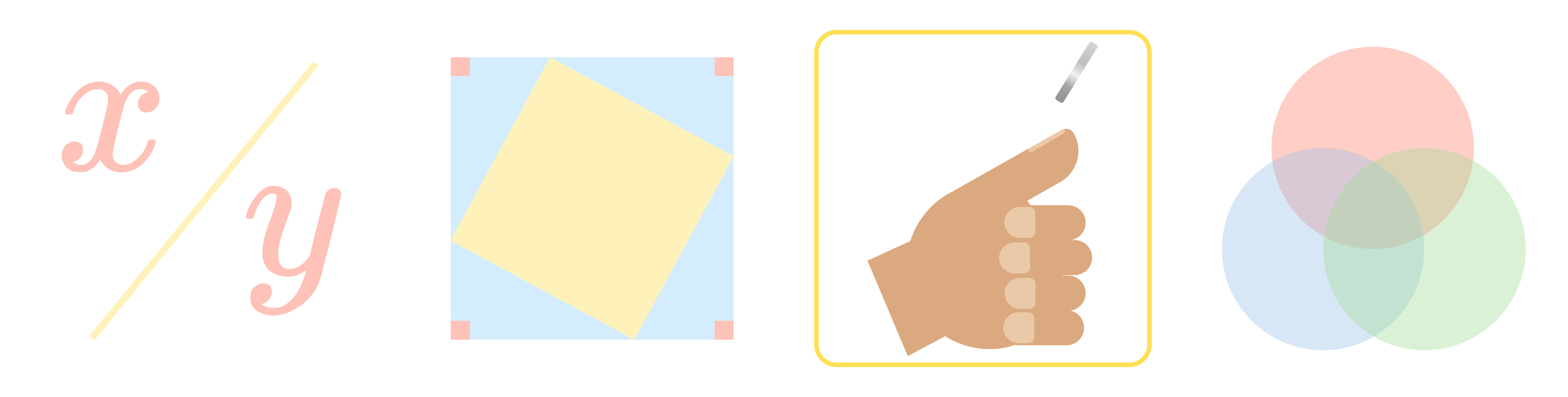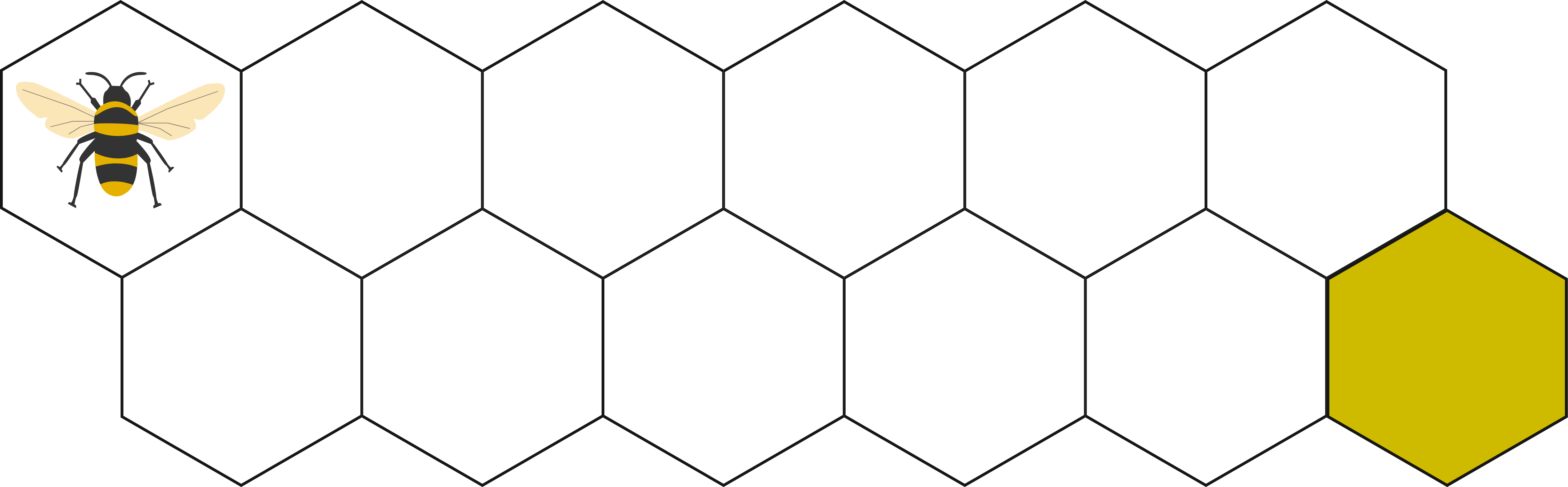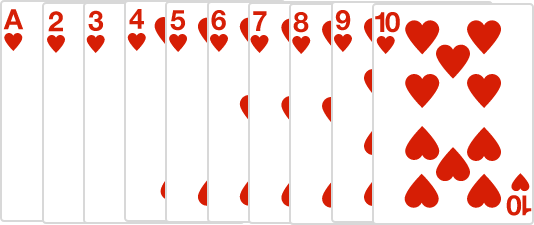### Contest Math ICombinatorics is the study of counting. Mathematicians who study combinatorics develop techniques to count outcomes, arrangements, and combinations of objects. These counting strategies can be applied to many different areas in mathematics, like probability, algebra, and geometry.

Competitive combinatorics problems often present situations that appear overwhelming and chaotic at first. To avoid being overwhelmed, it is important to focus on ways to organize the objects being counted. Look for ways to “simplify” the number of things you need to count: separate them into cases, focus only on the ones that meet certain criteria, and look for patterns.

# Combinatorics

A standard deck with 52 cards—26 red and 26 black—is shuffled.

What is the probability that the top card is the same color as the bottom card?# Combinatorics

How many ways are there to arrange the letters in the word "FLOOD" such that the two O's are not next to each other?# Combinatorics

Bombus the bumblebee wants to move from the top-left cell to the bottom-right cell containing honey.

Each time he moves, he can only take a step to a neighboring cell that moves him to the right.

How many ways does he have of reaching the honey in the last cell?Hint: Begin by finding the number of ways to reach some of the first few tiles.

# CombinatoricsIf I randomly pick a card from the ten above, the probability of it having an odd number or an even number is equal (an ace has a value of 1).

If I instead randomly pick two distinct cards from the ten above, does the sum of the two numbers have a higher probability of being odd or even?

# Combinatorics

The combinatorics questions in math competitions frequently focus on counting complicated arrangements and calculating the probability of events. We have units on each of these categories in this course, and you don't need to work through all of the units in this course in order, you can jump directly to whichever one you want to practice.

Our unit on Counting will teach you several visual and arithmetic tricks for organizing and counting objects in a variety of ways. To learn more, you can either explore what's in this unit or read through the Combinatorics: Counting Syllabus.

Our unit on Probability will show you how to apply these counting strategies to determine the real-life probability of events. It also delves into more complicated ways to select objects, including ways to calculate all possible combinations that can be selected from a group. To learn more, you can either explore what's in this unit or read through the Combinatorics: Probability Syllabus.

×